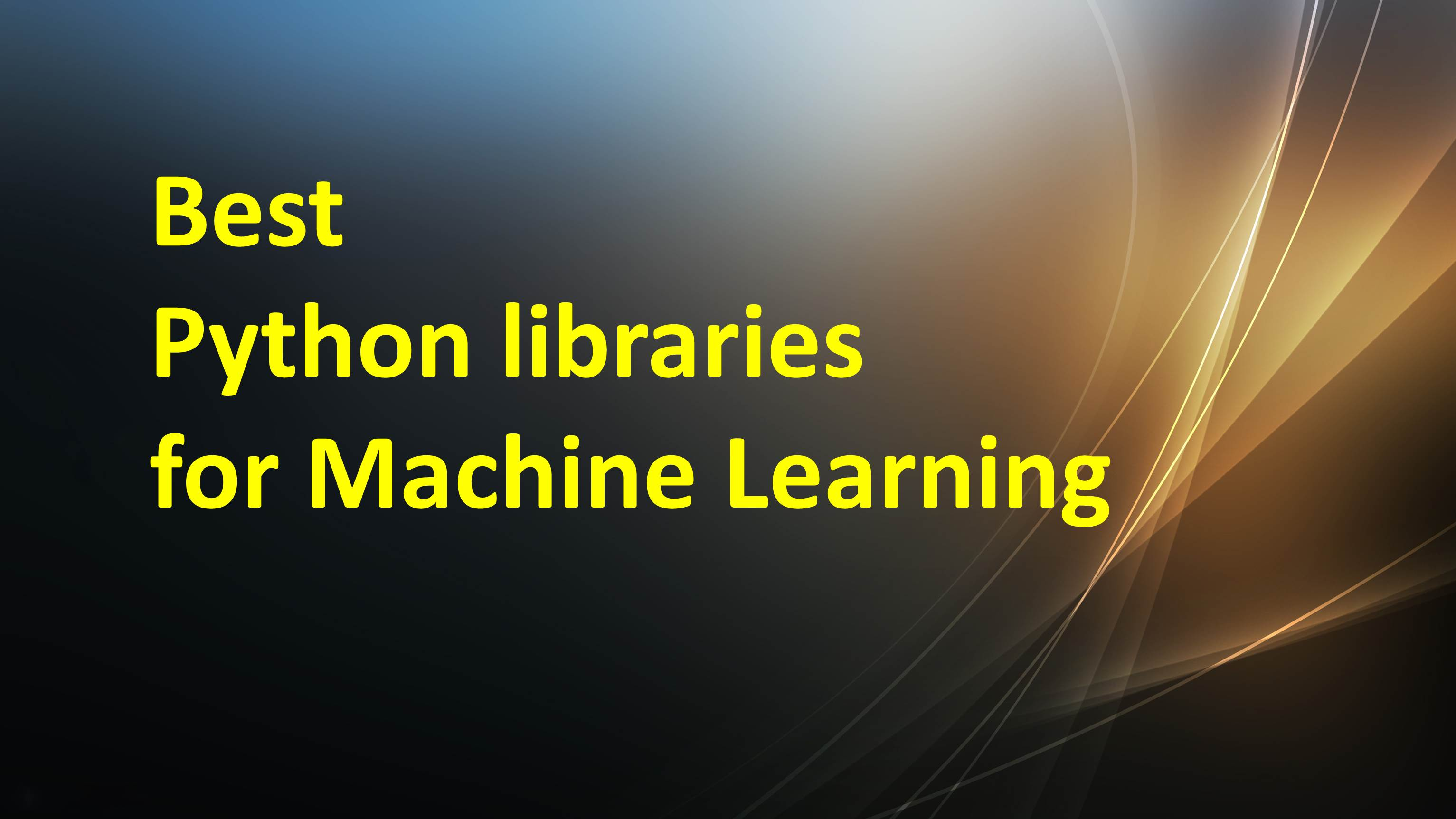/  Technology   /  Best Python libraries for Machine Learning## Best Python libraries for Machine Learning

Machine Learning, is the science of programming a computer by which they are able to learn from dissimilar kinds of data. According to Arthur Samuel is – “Machine Learning is the field of study that gives computers the ability to learn without being explicitly programmed.”

In earlier days people used to perform Machine Learning tasks by coding all the algorithms manually which was time consuming, tedious and ineffective. In this technology driven world it has become very much easy and efficient with various python libraries, frameworks, and modules.

Python is one of the most popular programming languages for this task and it has replaced many languages. One of the reasons is its vast collection of libraries. Let’s explore few Python libraries that are used in Machine Learning:

• Numpy
• Scipy
• Scikit-learn
• Theano
• TensorFlow
• Keras
• PyTorch
• Pandas
• Matplotlib

#### Numpy

NumPy is a well-known general-purpose array-processing package. High complex mathematical functions makes this library so powerful. Linear algebra, Fourier transforms, and random numbers can be handled by Numpy. Other high-end libraries like TensorFlow uses NumPy at the backend for manipulating tensors.

#### SciPy

The SciPy library offers modules for linear algebra, image optimization, integration interpolation, special functions, Fast Fourier transform, signal and image processing, Ordinary Differential Equation (ODE) solving, and other computational jobs in science and analytics.

Multi-dimensional array provided by the NumPy module is the underlying data structure used by SciPy.

#### Scikit-learn

NumPy and SciPy has become the most popular Python machine learning libraries for developing machine learning algorithms.  Skikit-learn is one of the well-known ML libraries for classical ML algorithms. It is built on top of two basic libraries, viz., NumPy and SciPy.

Scikit-learn algorithms that works on a consistent interface in Python.

Classification, regression, clustering, dimensionality reduction, model selection, and preprocessing are the major machine learning functions that the Scikit-learn library can handle.

#### Theano

Theano is built on NumPy and it exhibits a tight integration with NumPy. This can be implemented on Graphics Processing Unit (GPU) and CPU.  It is broadly used for unit-testing and self-verification to detect and diagnose various types of errors. It is used to define, evaluate and optimize mathematical expressions.

#### TensorFlow

Google Brain team developed Tensorflow. Its first release came in November 2015 under Apache License 2.0. Tensorflow is a popular framework for creating machine learning models. It involves defining and running computations involving tensors. This is widely used in the field of deep learning research and application.

#### Keras

Keras is an open-source library widely used for neural networks and machine learning. This can run on top of TensorFlow. This library can work efficiently on CPU and GPU. It works with neural-network building blocks like layers, objectives, activation functions, and optimizers. One of the best information about Keras is that it allows for easy and fast prototyping.

#### PyTorch

PyTorch has an extensive range of tools and libraries that supports on Computer Vision, Natural Language Processing(NLP) and many more ML programs.This library is open-source and is based on the Torch library. This library is easy to learn and easy to use. It has a robust framework to build computational graphs. The most useful thing of this is that it includes multi GPU support, simplified preprocessors, and custom data loaders.

#### Pandas

Pandas are turning up to be the most popular Python library. It is designed to work on both “relational” or “labeled” data and has wide variety of tools for data analysis and provides high-level data structures. This library uses 1-Dimensional and 2-Dimensional data structures.

#### Matplotlib

Matplotlib is a very popular 2D plotting data visualization library. It provides a high-quality image plots and figures in a variety of formats. You can easily generate histograms, plots, error charts, scatter plots, bar charts with just a few lines of code. Pyplot module makes it easy for programmers for plotting as it provides features to control line styles, font properties, formatting axes, etc.